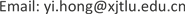FINFinance2161-0967Scientific Research Publishing10.12677/FIN.2019.91007FIN-28634FIN20190100000_88773879.pdf经济与管理 中国股票多头私募证券投资基金的风险因子研究 Empirical Study on Risk Factors for Long-Only Equity Hedge Funds in China 21文骜213*上海交通大学中国金融研究院，上海西交利物浦大学数学科学系，江苏 苏州null0701201909015873© Copyright 2014 by authors and Scientific Research Publishing Inc. 2014This work is licensed under the Creative Commons Attribution International License (CC BY). http://creativecommons.org/licenses/by/4.0/

1上海交通大学中国金融研究院，上海

2西交利物浦大学数学科学系，江苏 苏州Copyright © 2019 by author(s) and Hans Publishers Inc.1. 引言

2. 数据2.1. 私募证券投资基金样本数据

Sample statistics of private equity funds with investment strategie

Descriptive statistics of performance of private equity fund

2.2. 因子类型与构造

Factor types and definition

HS300股息率沪深300股息收益率Wind

2.2.1. Log变化率

log ( r e t t ) = log ( r e t t ) = log ( x t x t − 1 ) (1)

2.2.2. 信用风险指标

C s p r d t = log ( C B I n d e x t A A C B I n d e x t − 1 A A ) − log ( C B I n d e x t A A A C B I n d e x t − 1 A A A ) (2)

2.2.3. 封闭基金折价指标

C e f d i s r a t e = ∑ 1 n v o l u m e n * d i s c o u n t r a t e n t o t a l v o l u m e (3)

2.2.4. Pastor-Stambaugh流动性指标

r i , d + 1 , t e = a i , t + b i , t r i , d , t + c i , t s i g n ( r i , d , t e ) v i , d , t + є i , d + 1 , t (4)

2.2.5. 新开户数量变化率

(5)

Descriptive statistics of factor

HS300全收益指数0.00180.0677−0.01902.2313−0.23620.2296

HS300股息率0.00190.00312.01323.19630.00000.0138

3. 研究方法3.1. 研究方案设计

3.2. 回归模型介绍3.2.1. OLS回归模型

Ordinary Linear Squared，最小二乘法是以优化

min { 1 N | | y − X β | | 2 2 } (6)

y t = a + ∑ k = 1 n b k x k t + ε t (7)

3.2.2. Stepwise回归模型

A I C = 2 k − 2 ln ( L ) (8)

3.2.3. LASSO回归模型

1) LASSO回归模型描述

LASSO即最小绝对值收敛和选择算子(Least absolute shrinkage and selection operator)，是一种同时进行特征选择和正交化的回归分析方法。最初由斯坦福大学统计学教授Robert Tibshirani (1996)  于1996年基于Leo Breiman的非负参数推断(Nonnegative Garrote, NNG)提出。相比于普通线性回归模型(OLS或GLS)，在应对可能存在多重共线性的数据集时，其预测准确性和可解释性有一定增强。附录A详细介绍LASSO回归模型。

2) 利用LASSO模型选取变量子集进行OLS回归

3.3. 线性回归模型的误差调整

y t = a ˜ + ∑ k = 1 k b ˜ k x ˜ k t + ε ˜ t (9)

4. 实证研究结果及分析4.1. 模型解释度

Model Adj R2 statistic

OLS0.52860.57120.9267−0.2437
Stepwise0.57800.61020.93480.0000
LASSO (subset)0.53490.58930.9351−0.0286

4.2. 不同基金的风险因子差异

Statistics of selected factors with three models (significant factors in parenthesis

OLS13 (3.46)13 (3)13 (11)13 (0)
Stepwise4.94 (3.18)5 (3)11 (10)0 (0)
LASSO(subset)5.07 (2.74)4 (3)13 (9)0 (0)

4.3. 不同风险因子的解释力差异

4.4. 鲁棒性检验

5. 结语